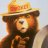# Is there Fibonachi Arcs indicator for ThinkorSwim?

A

#### alexguate

##### New member
Hello! I'm wondering if anyone has an automatic study script for a fibonacci Arcs, Fibonacci Spirals or a piece of code that it can get me start on it?
I'd appreciate it so much, Thank you!

Last edited:
T

#### tomsk

##### Well-known member
VIP
To the best of my knowledge there is no ThinkScript study developed for arcs/spirals, those are most likely from the Drawing Tools.
However I have seen somewhere some code posted for fibonacci fans.

A

#### alexguate

##### New member
To the best of my knowledge there is no ThinkScript study developed for arcs/spirals, those are most likely from the Drawing Tools.
However I have seen somewhere some code posted for fibonacci fans.
Hi, I'm sorry for posting it twice (a beginners mistake) I've seen someone with a script which display arcs but sadly it was too expensive. I have the fibonacci Fan script and I've been trying to convert the Fib Fans to Fib Arcs but sadly no progress so far. Thank you for the reply!

S

#### Samus Aran

##### New member
I'm extremely interested in one too. I'm willing to paid someone in the forum to help me finish the bugs on my automated Fibonacci Arc study script.

\$100 offer to help me finish an automated study script for Fibonacci Arcs plus I'll post the finish version here for everyone!

Code:
``````# Objective: to make my own Fibonacci Arc indicator for the purpose of only Exiting my trades with no human input,  meaning "automated" the Fibonacci Arc provided in the drawing tools cannot be access in code meaning I cannot automate my trading, so I have to code one myself to myself.

#----->Start of Slope Calculations to determine only if the trend is Upward or Downward and nothing else <-----
def a = HighestAll(high);
def b = LowestAll(low);

def barnumber = barNumber();
def c = if high == a then barnumber else double.nan;
def d = if low == b then barnumber else double.nan;
rec highnumber = compoundValue(1, if IsNaN(c) then highnumber else c, c);
def highnumberall = HighestAll(highnumber);
rec lownumber = compoundValue(1, if IsNaN(d) then lownumber else d, d);
def lownumberall = LowestAll(lownumber);

def upward = highnumberall > lownumberall;
def downward = highnumberall < lownumberall;

def x = AbsValue(lownumberall - highnumberall );

def slope = (a - b) / x;
def slopelow = (b - a) / x;

def line = b + (slope * (barnumber - lownumber));
def linelow = a + (slopelow * (barnumber - highnumber));
def currentlinelow = if barnumber <= lownumberall then linelow else double.nan;
def currentline = if barnumber <= highnumberall then line else double.nan;

#----->END<-----

#----->Start of drawing a circle around the high close price if the Trend is Upward or a circle on the lowest point close price if the Trend is Downward and nothing else <-----
def r = ((1.618)/(highnumberall)) ;
plot Arc1 = fold i = 0 to 360 while i != 360 do ( highnumberall ) +  (i*((r*2*double.Pi)/(360)))  and ( highnumberall ) - (i*((r*2*double.Pi)/(360)));
Arc1.SetDefaultColor(Color.DARK_GREEN);
Arc1.SetStyle(Curve.FIRM);

def  r2 = ((23.6)/(lownumberall));
plot Arc2 = fold i2 = 0 to 360  while i2 != 360 do ( lownumberall ) - i2*((r2*2*double.Pi)/(360)) and ( lownumberall ) + (i2*((r2*2*double.Pi)/(360))) ;
Arc2.SetDefaultColor(Color.pINK);
Arc2.SetStyle(Curve.FIRM);

#----->END<-----``````Midpoint Moving Average Indicator Questions 2